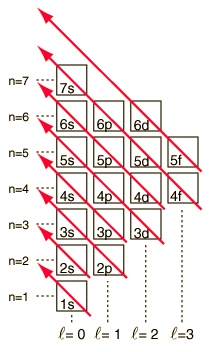# Order of Filling of Electron StatesAs the periodic table of the elements is built up by adding the necessary electrons to match the atomic number, the electrons will take the lowest energy consistent with the Pauli exclusion principle. The maximum population of each shell is determined by the quantum numbers and the diagram at left is one way to illustrate the order of filling of the electron energy states. For a single electron, the energy is determined by the principal quantum n number and that quantum number is used to indicate the "shell" in which the electrons reside. For a given shell in multi-electron atoms, those electrons with lower orbital quantum number l will be lower in energy because of greater penetration of the shielding cloud of electrons in inner shells. These energy levels are specified by the principal and orbital quantum numbers using the spectroscopic notation. When you reach the 4s level, the dependence upon orbital quantum number is so large that the 4s is lower than the 3d. Although there are minor exceptions, the level crossing follows the scheme indicated in the diagram, with the arrows indicating the points at which one moves to the next shell rather than proceeding to higher orbital quantum number in the same shell. The electron configuration for any element may be found by clicking on that element in the periodic table. The first exception to the above scheme that is encountered is chromium, where the fifth 3d electron state is occupied instead of the second 4s state.

 See another approach to the order of filling
Index

Reference
Rohlf
Sec. 9-3.

 HyperPhysics*****Chemistry R Nave
Go Back

# Order of Filling of Electron StatesAs the periodic table of the elements is built up by adding the necessary electrons to match the atomic number, the electrons will take the lowest energy consistent with the Pauli exclusion principle. The maximum population of each shell is determined by the quantum numbers and the diagram above is one way to illustrate the order of filling of the electron energy states.

For a single electron, the energy is determined by the principal quantum n number and that quantum number is used to indicate the "shell" in which the electrons reside. For a given shell in multi-electron atoms, those electrons with lower orbital quantum number l will be lower in energy because of greater penetration of the shielding cloud of electrons in inner shells. These energy levels are specified by the principal and orbital quantum numbers using the spectroscopic notation. When you reach the 4s level, the dependence upon orbital quantum number is so large that the 4s is lower than the 3d. The nature of the level crossing follows the dashed line in the diagram, which shows the points at which one moves to the next shell rather than proceeding to higher orbital quantum number in the same shell.

The electron configuration of most elements can be implied from the above diagram, but not all. The electron configuration for any element may be found by clicking on that element in the periodic table. The first exception to the above scheme that is encountered is chromium, where the fifth 3d electron state is occupied instead of the second 4s state. Copper is also an exception, filling all 10 3d states rather than the second 4s state. Niobium fills the 4th 4d state before filling the second 5s state.

Index

Reference
Pauling
College Chemistry

Halliday & Resnick

 HyperPhysics*****Chemistry R Nave
Go Back